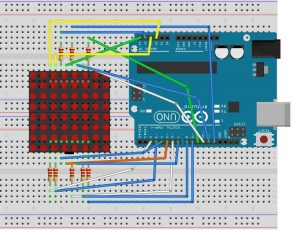21.02.2016

# Lesson #5 – Use a LED-Matrix

In this lesson we’re going to let a LED-Matrix showing a Smiley.

For that do we need:

• 1 Arduino
• 1 LED-Matrix 8×8 (e.g. SH1388ASR with common cathode or 1588BS with common anode)
• 8 220Ω Resistors
• 16 Jumper

We have to tell the Arduino, on which pins the rows and columns have been connected. To make it in a simple way, we define an array and a for-loop, which defines the Arduino pins.

An array with content will be defined like this:

var_type var_name[size] = {val_0, val_1, val_2, val_n};

e.g.: int rows = {10,18,9,13,2,8,3,6};

The `const` before the variable type defines a constant variable. It helps the compiler to to compile the sketch in a better way.

You use the for-loop like this:

for(init counter; test counter; increment OR decrease counter)
{
//program code
}

For example (do things eight times, from zero to eight):

for(int i = 0; i < 8; i++)
{
//program code
}

That for-loop in a sentence means:

`For` an `int i` with `0`, while i` < 8`, increase i` plus one`, `do` the program code.

Now we have to define a two dimensional array for our smiley (1 = on, 0 = off). It’s the same like a normal array, just with to indexes:

var_type var_name[size_row] [size_column] = {
{val_0, val_1, val_2, val_n},  /*row 0*/
{val_0, val_1, val_2, val_n},  /*row 1*/
{…}
};

In the `loop()` method we have two for-loops in each other, to jump from row to row and from column to column.

```//Pins, connected to the rows (220 Ohm resisor)
const int rows = {10,18,9,13,2,8,3,6};
// Pins, connected to the columns
const int columns = {19,4,5,11,7,12,17,16};

int smiley = {
{0,1,1,0,0,1,1,0},
{0,1,1,0,0,1,1,0},
{0,0,0,0,0,0,0,0},
{0,0,0,1,1,0,0,0},
{0,0,0,1,1,0,0,0},
{0,1,0,0,0,0,1,0},
{0,0,1,0,0,1,0,0},
{0,0,0,1,1,0,0,0}};

void setup()
{
Serial.begin(9600);
//set the pins to output
for (int pin = 0; pin < 8; pin++)
{
pinMode(rows[pin], OUTPUT);
pinMode(columns[pin], OUTPUT);
//all LEDs off
digitalWrite(rows[pin], LOW);
digitalWrite(columns[pin], LOW);
}

}

void loop()
{
for (int row = 0; row < 8; row++)
{
for (int column = 0; column < 8; column++)
{
digitalWrite(rows[row],HIGH);
digitalWrite(columns[column], !(smiley[row][column]));
digitalWrite(rows[row],LOW);
digitalWrite(columns[column],HIGH);
}
}
}```

To see, what your code is doing, you have to build your circuit. Do it according to the following scheme:Source: http://mikrocontrollerkochbuch.de/index.php?title=Matrix-Modul_mit_8_x_8_LEDs_Version_1